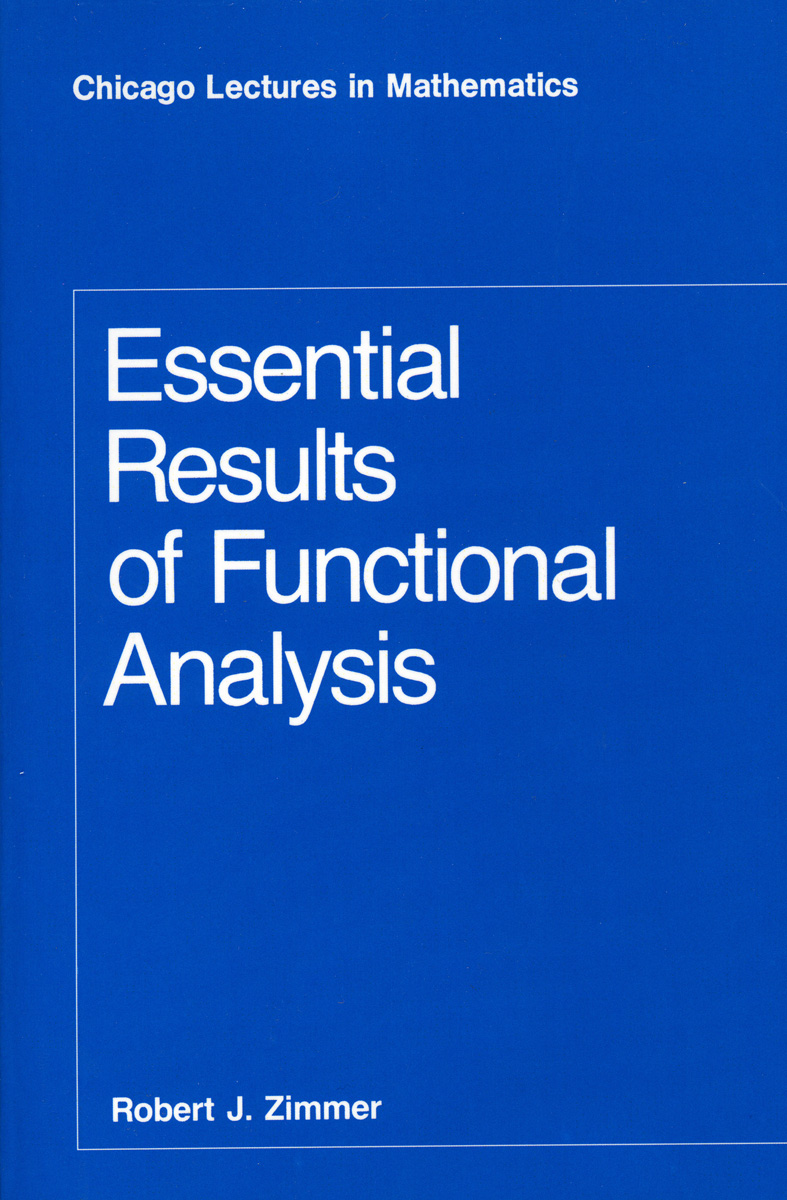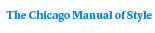# Essential Results of Functional Analysis

Robert J. Zimmer168 pages | 5.25 x 8 | © 1990
Paper \$28.00 ISBN: 9780226983387 Published January 1990
Functional analysis is a broad mathematical area with strong connections to many domains within mathematics and physics. This book, based on a first-year graduate course taught by Robert J. Zimmer at the University of Chicago, is a complete, concise presentation of fundamental ideas and theorems of functional analysis. It introduces essential notions and results from many areas of mathematics to which functional analysis makes important contributions, and it demonstrates the unity of perspective and technique made possible by the functional analytic approach.

Zimmer provides an introductory chapter summarizing measure theory and the elementary theory of Banach and Hilbert spaces, followed by a discussion of various examples of topological vector spaces, seminorms defining them, and natural classes of linear operators. He then presents basic results for a wide range of topics: convexity and fixed point theorems, compact operators, compact groups and their representations, spectral theory of bounded operators, ergodic theory, commutative C*-algebras, Fourier transforms, Sobolev embedding theorems, distributions, and elliptic differential operators. In treating all of these topics, Zimmer’s emphasis is not on the development of all related machinery or on encyclopedic coverage but rather on the direct, complete presentation of central theorems and the structural framework and examples needed to understand them. Sets of exercises are included at the end of each chapter.

For graduate students and researchers in mathematics who have mastered elementary analysis, this book is an entrée and reference to the full range of theory and applications in which functional analysis plays a part. For physics students and researchers interested in these topics, the lectures supply a thorough mathematical grounding.
Table of Contents• Contents
Contents
Preface
0. Background
0.A. Review of basic functional analysis
0.B. Some special properties of integration in Rn
1. Topological vector spaces and operators
1.1. Examples of spaces
1.2. Examples of operators
1.3. Operator topologies and groups of operators
2. Convexity and fixed point theorems
2.1. Kakutani-Markov fixed point theorem
2.2. Haar measure for compact groups
2.3. Krein-Millman theorem
3. Compact operators
3.1. Compact operators and Hilbert-Schmidt operators
3.2. Spectral theorem for compact normal operators
3.3. Peter-Weyl theorem for compact groups
4. General spectral theory
4.1. Spectrum of an operator
4.2. Spectral theorem for self-adjoint operators
4.3. Gelfand’s theory of commutative C*-algebras
4.4. Mean ergodic theorem
5. Fourier transforms and Sobolev embedding theorems
5.1. Basic properties of the Fourier transform and the Plancherel theorem
5.2. Sobolev and Rellich embedding theorems
6. Distributions and elliptic operators
6.1. Basic properties of distributions
6.2. Distributions and Sobolev spaces
6.3. Regularity for elliptic operators
6.4. Appendix to 6.3: proof of Garding’s inequality
6.5. A spectral theorem for elliptic operators
Index
For more information, or to order this book, please visit https://www.press.uchicago.edu